# Matplotlib Pyplot

The `pyplot` object is the main workhorse of matplotlib library. It is through pyplot that you can create the figure canvas, various types of plots, modify and decorate them.

## Contents

1. Pyplot: Basic Overview
2. General Functions in pyplot
3. Line plot
4. Scatter plot
5. Pie chart
6. Histogram
7. 2D Histograms
8. Bar plot
9. Stacked Barplot
10. Boxplot
11. Stackplot
12. Time series plotting
13. Removing title overlaps in multiplots
14. Irregular plot layout using GridSpec

## 1. Pyplot – Basic Overview

Matplotlib library in python has a dual interface:

1. A MATLAB style interface which makes graphs and visualisations like MATLAB
2. An Object Oriented Interface.

The MATLAB-style tools are contained in the `pyplot` interface which is often imported as `plt`.

The object oriented interface becomes useful when we get into complicated situations.

Like if you create 2 plots in the same figure, then you wont be able to alter changes to the first graph once you have created the second graph in MATLAB interface, but this is possible using object oriented inteface.

The `pyplot` provides the `matlab` like way of plotting functionality. That is, the plotting functions that you call with `pyplot` will be incrementally applied on the same currently active plot (`subplot`).

Now lets look into `pyplot` in detail.

First, import the required libraries

``````
import numpy as np
import pandas as pd
import matplotlib.pyplot as plt
%matplotlib inline
plt.rcParams.update({'figure.figsize':(10,7.5), 'figure.dpi':100})

/* if this shows error first you have to install the matplotlib library, using pip:
!pip install matplotlib */``````

The line `%matplotlib inline` will enable interactive plots embedded with the jupyter notebook and the likes.

`plt.rcParams.update()` changes the default parameters in matplotlib applying the properties to all the plots created in the notebook.

I will use an existing Datset – House Prediction Dataset.

## 2. Pyplot functions you must know

The `pyplot` object is the main workhorse of matplotlib library. It is through pyplot that you can create a figure, create various types of plots, modify and decorate them. Even modifying the individual components of plots is achieved through `pyplot` only.

The commonly used functions in `pyplot` is below. By knowing the usage of these commands, you will be able to create pretty much any type of customization you want. A more detailed structured walkthrough of these pyplot commands is written in the matplotlib tutorial.

#### IMPORTANT PYPLOT COMMANDS YOU MUST KNOW

1. `plt.figure()` – Set the properties of the figure canvas, like `figsize`, `dpi` etc.
2. `plt.subplot()` – Select the subplot to draw within the figure.
3. `plt.plot()` – Makes a line plot by default. Can make other types
4. `plt.subplots()` – Create and initialize the figure and axes. This outputs the figure and axis objects.
5. `plt.scatter(x,y)` – Draw a scatterplot of x and y
6. `plt.pie()` – Create a pie chart
7. `plt.hist()` – Create a histogram
8. `plt.hist2d()` – Create 2-D Histogram
9. `plt.bar()` – Create bar plot
10. `plt.text()` – Add text annotation inside the plot
11. `plt.colorbar()` – Add a colorbar. Used when color of dots in scatterplot is set based on a continuous numeric variable.
12. `plt.xlabel(), plt.ylabel()` – label the x and y axis
13. `plt.xlim(), plt.ylim()` – Set X and Y axis limits
14. `plt.title()` – Add current plot’s title
15. `plt.legend()` – Set the legend for current plot
16. `plt.suptitle()` – Apply a title for entire figure
17. `plt.tight_layout()` – Tightly arrange the subplots
18. `plt.xticks()`, `plt.yticks()` – Adjust the x and y axis ticks position and labels
19. `plt.gca()`, `plt.gcf()` – Get the current axis and figure
20. `plt.subplot2grid` and `plt.GridSpec` – Lets you draw complex layouts
21. `plt.show()` – Display the entire plot
22. `plt.rcParams.update()` – Update global plotting parameters

Now lets look into the common types of graphs we can plot using pyplot.

## 3. Line Plots

Use the ‘ plt.plot(x,y) ‘ function to plot the relation between x and y.

I created an Artificial Dataset using the `np.linspace()` command.

We need to specify the lower limit, upper limit and the no of points required.

``````/* Simple lines plot */
x = np.linspace(0,5,50) # 50 values between 0 and 5.
y1 = np.exp(x)
y2 = np.exp(x-1)
y3 = np.exp(x+1)

plt.plot(x,y1, '-',color='blue')
plt.plot(x,y2,'--',color='red')
plt.plot(x,y3, '-c',color='green');
plt.xlabel('X Axis')
plt.ylabel('Exponetial value')
plt.show()
/* '--' generates line graph with dashed lines */
``````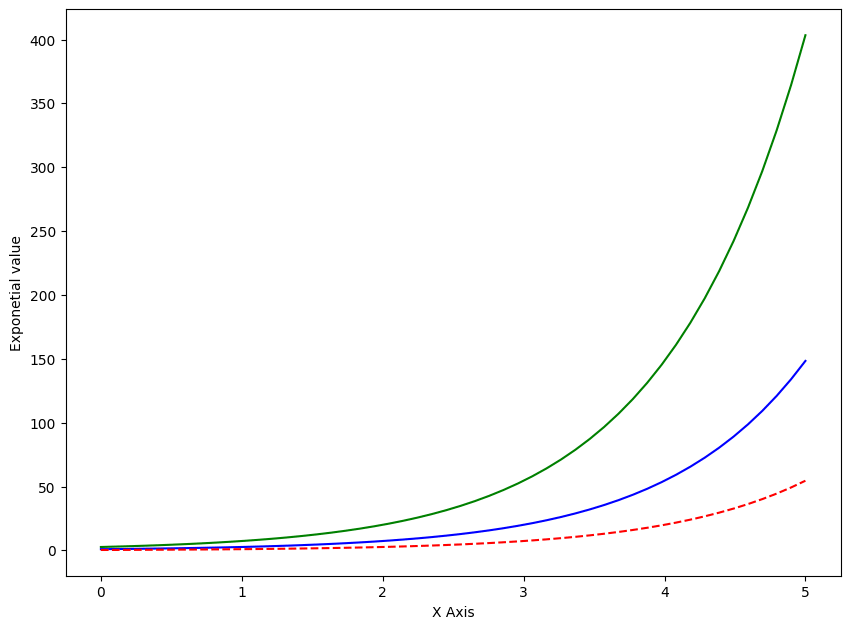## 4. Scatter Plots

1) Use `plt.plot()` function to draw simple scatter plots, you just need to specify `'o'` inside the `plt.plot()` function. This will place dots in the chart. Specifying a `*` will place that character.

2) You can use `plt.scatter()` function also to plot the scatter plot.

The difference between using `plt.plot()` vs `plt.scatter()` to make a scatterplot is, `plt.scatter()` provides more flexibility to modify the color, shape and size of each dot. This is not possible with `plt.plot()`

``````/* Scatterplot with plt.plot vs plt.scatter */
/* Data */
x = np.linspace(0,5,20)
y1 = np.sin(x)
y2 = np.sin(x+1)

/* Plot */
plt.plot(x,y1,'o',color='blue', label='plt.plot')
plt.scatter(x, y2, c=y2, label='plt.scatter', cmap='Reds')

/* Decorate */
plt.title('Scatterplot with plt.plot vs plt.scatter')
plt.xlabel('x- VALUE')
plt.ylabel(' sine function ')
plt.legend(loc='upper right')
plt.colorbar()
plt.show()``````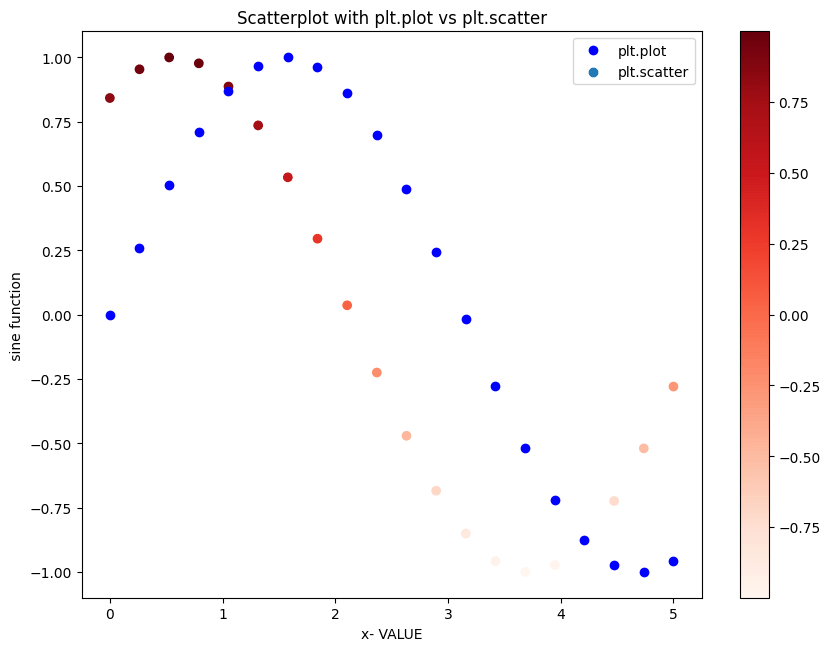You can see that both the functions give exactly the same results.

## Colormaps

In above chart, the points took a gradient of red colors because, I’d specified the `cmap='Reds'` inside `plt.scatter()`.

Matplotlib comes with a large collection of such colormap palettes by default and you see all of them using `dir(plt.cm)`.

``print(dir(plt.cm))``
``['Accent', 'Accent_r', 'Blues', 'Blues_r', 'BrBG', 'BrBG_r', 'BuGn', 'BuGn_r', 'BuPu', 'BuPu_r', 'CMRmap', 'CMRmap_r', 'Dark2', 'Dark2_r', 'GnBu', 'GnBu_r', 'Greens', 'Greens_r', 'Greys', 'Greys_r', 'LUTSIZE', 'OrRd', 'OrRd_r', 'Oranges', 'Oranges_r', 'PRGn', 'PRGn_r', 'Paired', 'Paired_r', 'Pastel1', 'Pastel1_r', 'Pastel2', 'Pastel2_r', 'PiYG', 'PiYG_r', 'PuBu', 'PuBuGn', 'PuBuGn_r', 'PuBu_r', 'PuOr', 'PuOr_r', 'PuRd', 'PuRd_r', 'Purples', 'Purples_r', 'RdBu', 'RdBu_r', 'RdGy', 'RdGy_r', 'RdPu', 'RdPu_r', 'RdYlBu', 'RdYlBu_r', 'RdYlGn', 'RdYlGn_r', 'Reds', 'Reds_r', 'ScalarMappable', 'Set1', 'Set1_r', 'Set2', 'Set2_r', 'Set3', 'Set3_r', 'Spectral', 'Spectral_r', 'Wistia', 'Wistia_r', 'YlGn', 'YlGnBu', 'YlGnBu_r', 'YlGn_r', 'YlOrBr', 'YlOrBr_r', 'YlOrRd', 'YlOrRd_r', '__builtins__', '__cached__', '__doc__', '__file__', '__loader__', '__name__', '__package__', '__spec__', '_gen_cmap_d', '_reverser', 'afmhot', 'afmhot_r', 'autumn', 'autumn_r', 'binary', 'binary_r', 'bone', 'bone_r', 'brg', 'brg_r', 'bwr', 'bwr_r', 'cbook', 'cividis', 'cividis_r', 'cmap_d', 'cmaps_listed', 'colors', 'cool', 'cool_r', 'coolwarm', 'coolwarm_r', 'copper', 'copper_r', 'cubehelix', 'cubehelix_r', 'datad', 'flag', 'flag_r', 'functools', 'get_cmap', 'gist_earth', 'gist_earth_r', 'gist_gray', 'gist_gray_r', 'gist_heat', 'gist_heat_r', 'gist_ncar', 'gist_ncar_r', 'gist_rainbow', 'gist_rainbow_r', 'gist_stern', 'gist_stern_r', 'gist_yarg', 'gist_yarg_r', 'gnuplot', 'gnuplot2', 'gnuplot2_r', 'gnuplot_r', 'gray', 'gray_r', 'hot', 'hot_r', 'hsv', 'hsv_r', 'inferno', 'inferno_r', 'jet', 'jet_r', 'ma', 'magma', 'magma_r', 'mpl', 'nipy_spectral', 'nipy_spectral_r', 'np', 'ocean', 'ocean_r', 'pink', 'pink_r', 'plasma', 'plasma_r', 'prism', 'prism_r', 'rainbow', 'rainbow_r', 'register_cmap', 'revcmap', 'seismic', 'seismic_r', 'spring', 'spring_r', 'summer', 'summer_r', 'tab10', 'tab10_r', 'tab20', 'tab20_r', 'tab20b', 'tab20b_r', 'tab20c', 'tab20c_r', 'terrain', 'terrain_r', 'twilight', 'twilight_r', 'twilight_shifted', 'twilight_shifted_r', 'viridis', 'viridis_r', 'winter', 'winter_r']``

## 5. Pie Chart

Pie chart shows the distribution of data based on the proportion of the pie occupied.

Use `plt.pie()` command to plot pie chart in python.

``````/* Pie Chart (exploded) */
explode = [0.1,0.1,0.15,0.1,0.15]

/* explode function is used to show each pie separately */
x = ['LabelA','LabelB','LabelC','LabelD','LabelE']
y = [200,250,1000,750,900]
plt.pie(y,
labels= x,
colors = ['red', 'orange','darkorange','blue','yellow'],
explode=explode);``````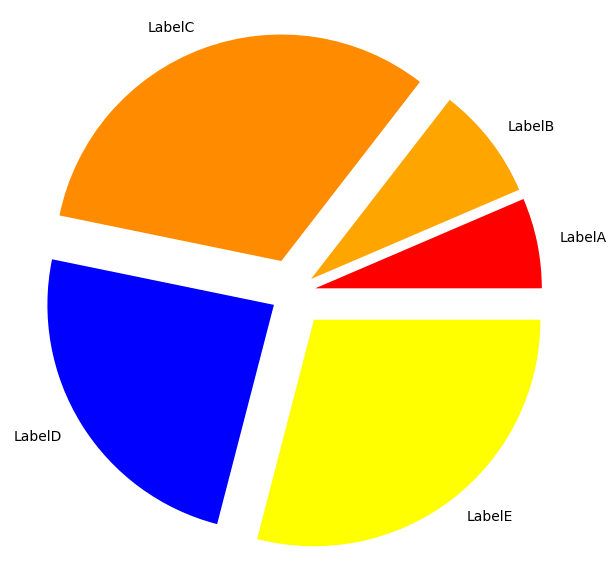## 6. Histogram

`plt.hist()` command is used to draw histogram in matplotlib.

You need to specify the array and the no of bins as input to the function. See more histogram examples.

For this let’s use the house dataset from kaggle.

``````
/* Import Data */
url = 'house.csv'
url = 'house.csv'
``````
/* Multiple Histograms */
/* Data */
df1 = df[df['SaleCondition']=='Normal']
df2 = df[df['SaleCondition']=='Abnorml']
df3 = df[df['SaleCondition']=='Partial']

/* Change the size of the plot */
plt.rcParams.update({'figure.figsize':(7.5,5), 'figure.dpi':100})

/* Plot the histogram for all 3 parts in the same graph */
plt.hist(df1['SalePrice'], bins=50)
plt.hist(df2['SalePrice'], bins=50)
plt.hist(df3['SalePrice'], bins=50)
plt.xlabel('Price')
plt.ylabel('Distribution')
plt.show()``````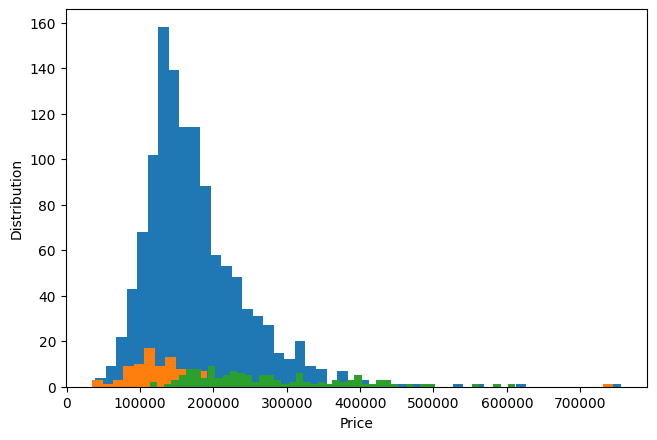## 7. 2-D Histogram

Use either the `plt.hist2d` or `plt.hexbin` function to draw the historgram.

First create a dataset using multivariate Gaussian Distribution.

``````/* 2D Histogram */
mean = [10, 10]
cov = [[1, 2], [2, 3]]
x, y = np.random.multivariate_normal(mean, cov, 1000).T
plt.hist2d(x, y, bins=100, cmap='Blues')
cb = plt.colorbar()``````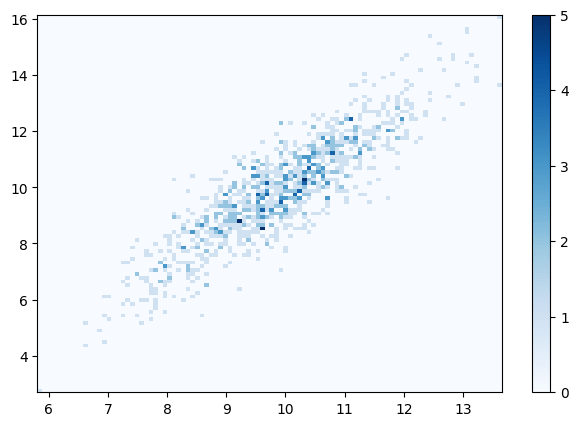## 8. Bar Plot

Use `plt.bar()` function to plot bar graph in matplotlib.

You need to specify the index(X axis) and height(Y axis) as arguments for the bar plot. See more bar plot examples.

``````/* Bar Chart */
url = 'house.csv'
sales = df[['SaleCondition', 'SalePrice']].groupby('SaleCondition').median()

/* Plot */
plt.bar(sales.index, height=sales.SalePrice, color='pink');``````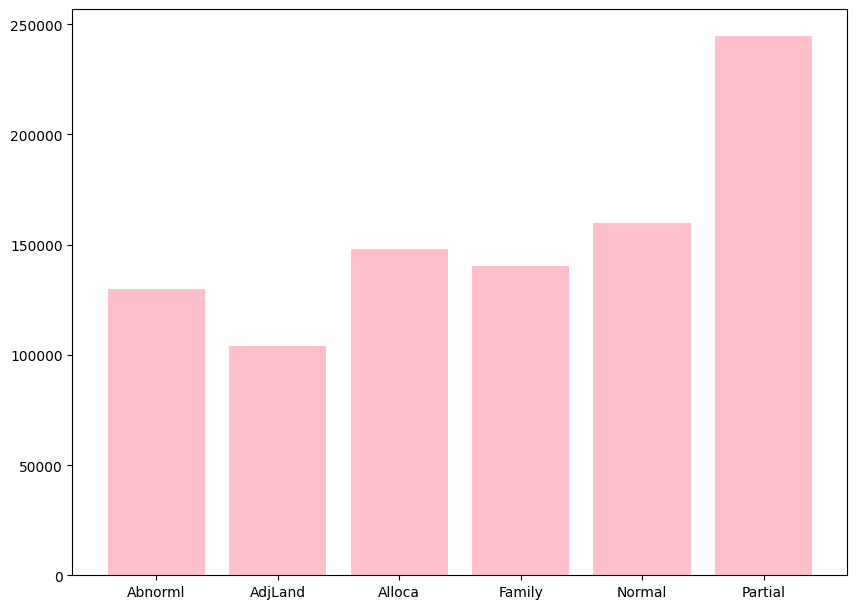## 9. Stacked Bar Plot

The stacked bar chart stacks bars that represent different groups on top of each other.

This can be done in pandas library by using `stacked='True'` command in `df.plot()` function.

``````
/* Stacked Bar Plot */
/* Data */
url = 'house.csv'
less     = df.loc[df['SalePrice']<200000, :]['SaleCondition'].value_counts()
greater  = df.loc[df['SalePrice']>200000, :]['SaleCondition'].value_counts()
df_plot  = pd.DataFrame([less, greater])
df_plot.index=['<50000','>50000']

/* Plot */
df_plot.plot(kind='bar',stacked=True, title='Stacked Bar plot');``````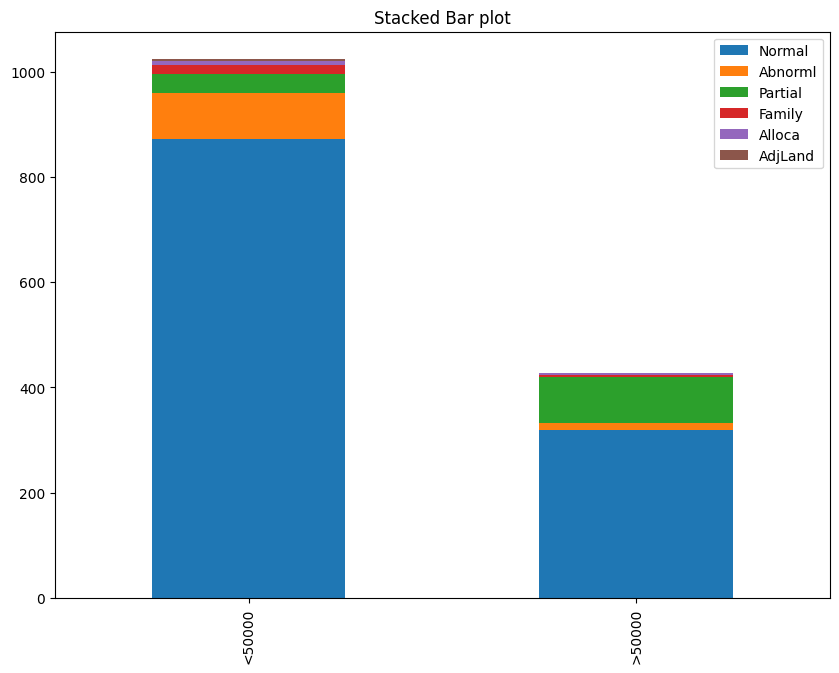## 10. Boxplot

Box plot helps in understanding the distribution more better.

You will be able to find the maximum value, minimum value and also the median value excluding the outliers in the data.

For plotting Boxplot, use `plt.boxplot()` function with x value as argument. See more boxplot examples.

``````/* Box Plot */
url = 'house.csv'

/* Plot */
plt.boxplot(df['SalePrice'],patch_artist=True, notch=True);``````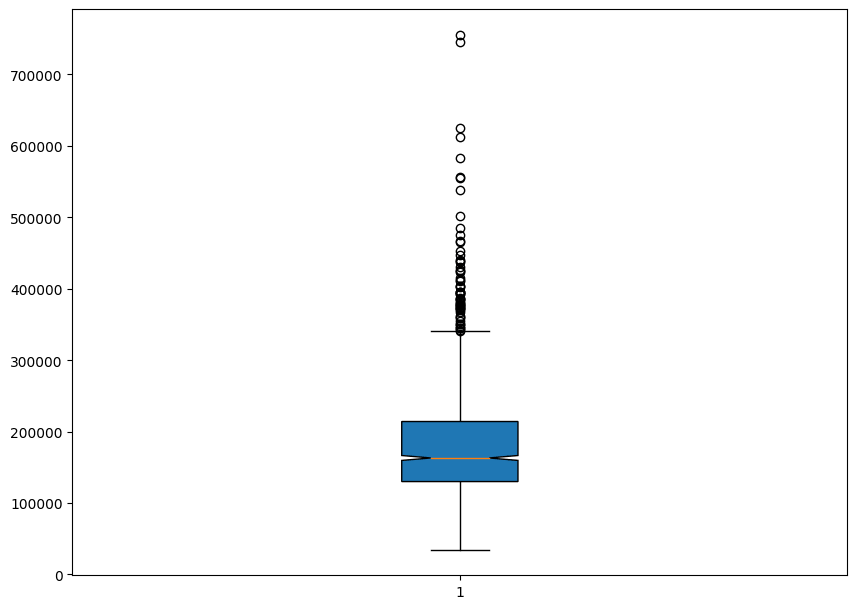The lower line represents the minimum value and the middle line represents the median value, the notch represents the 95% confidence interval and the top line represents the maximum value excluding the outliers.

## 11. Stackplot

A stackplot is a line chart that is subdivided into its components so that the proportional contributions, as well as the totals, can be seen.

Use the `stackplot()` function with x and y as arguments to plot the graph.

``````x = [0, 1, 2, 3]
y1 = [10, 15, 20, 10]
y2 = [0, 2, 8, 5]
y3 = [6, 20, 18, 14]
y = np.vstack([y1, y2, y3])
fig, ax = plt.subplots()
ax.stackplot(x, y1, y2, y3)
plt.show()``````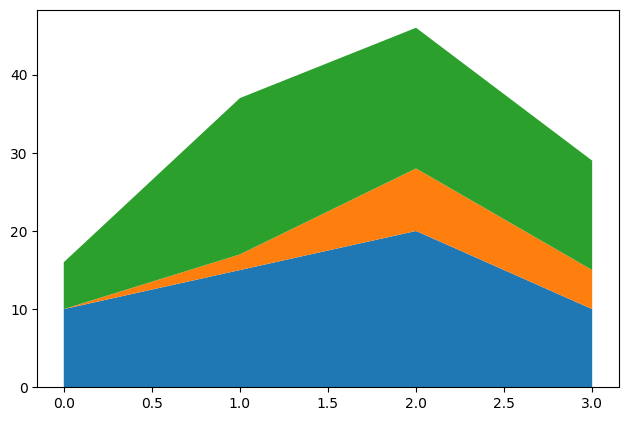## 12. Time Series Plotting

Use the same function `plt.plot()` which we used for line plot to plot the time series function.

Let’s use the candy production dataset.

``````df = pd.read_csv("candy_production.csv")
observation_date IPG3113N
0 1972-01-01 85.6945
1 1972-02-01 71.8200
2 1972-03-01 66.0229
3 1972-04-01 64.5645
4 1972-05-01 65.0100
``````/* Time Series Plot */
/* Plot */
plt.plot(df['observation_date'], df['IPG3113N'])

/* Decorate */
xtick_labels = pd.to_datetime(df['observation_date'][::48]).dt.strftime('%Y-%m')
plt.xticks(df['observation_date'][::48], xtick_labels, rotation=30)
plt.title('Candy Production');``````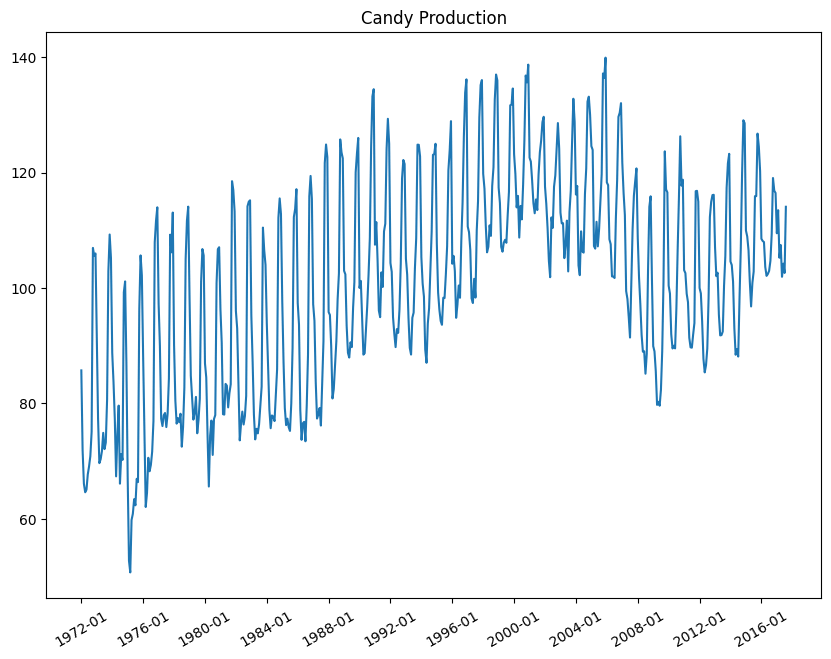You can also divide the dataset into 2 parts and visualize it into different colours.

``````/* Time Series Plot in multiple colors */
plt.plot(df['IPG3113N'][:100])
plt.plot(df['IPG3113N'][100:200])
plt.plot(df['IPG3113N'][200:300])
plt.plot(df['IPG3113N'][300:], color='black')
plt.title('Line Series plot with multiple colors');``````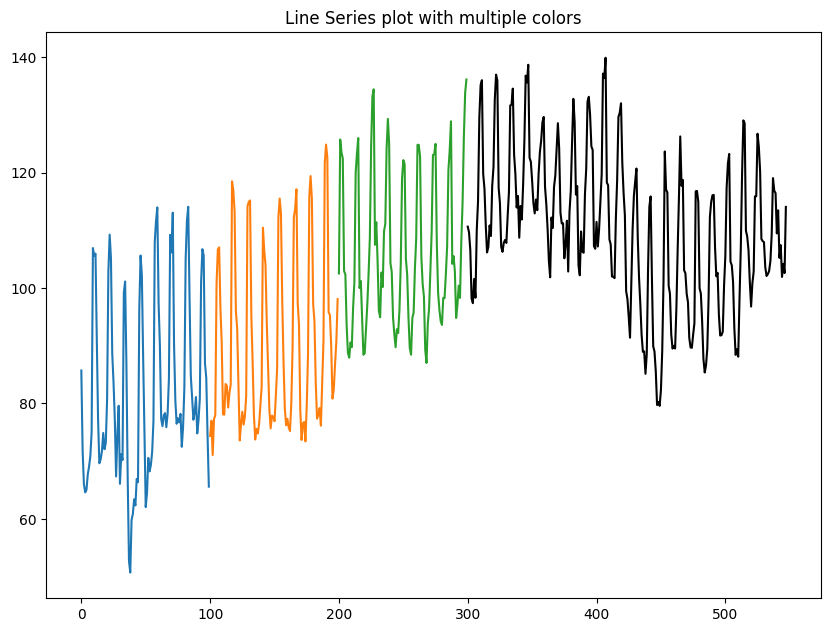## 13. Removing title overlaps in multiplots

`tight_layout` attempts to resize subplots in a figure so that there are no overlaps between axes objects and labels on the axes.

I will create 4 grids in the same plot initially without using the `tight_layout` function and then including the function to see the difference.

``````/* Plots with overlapping titles */
import matplotlib.pyplot as plt
plt.rcParams.update({'figure.figsize':(7.5,5), 'figure.dpi':100})

def plotnew(ax):
ax.plot([1,2])
ax.set_xlabel('x-label')
ax.set_ylabel('y-label')
ax.set_title('Title')

fig, ((ax1, ax2), (ax3, ax4)) = plt.subplots(nrows=2, ncols=2)
fig.suptitle('Plot with Ugly Overlapping titles')
plotnew(ax1)
plotnew(ax2)
plotnew(ax3)
plotnew(ax4)``````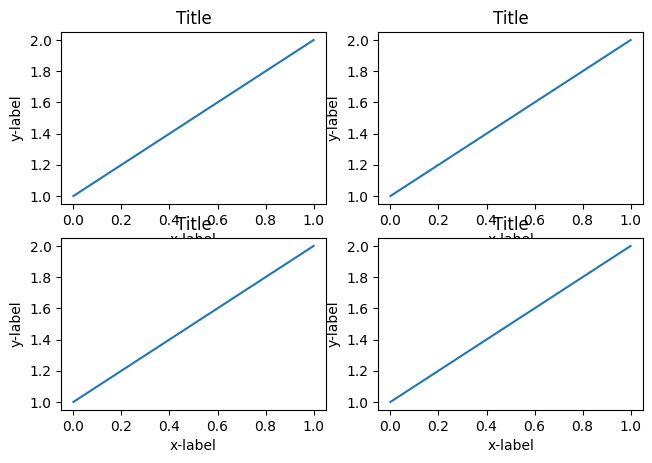You can see that in the above plot, there is overlap between the axis names and the titles of different plot.

For fixing this, use `tight_layout()` function to automatically arrange them nicely.

``````/* Non Overlapping Titles */
import matplotlib.pyplot as plt
plt.rcParams.update({'figure.figsize':(7.5,5), 'figure.dpi':100})
def plotnew(ax):
ax.plot([1,2])
ax.set_xlabel('x-label')
ax.set_ylabel('y-label')
ax.set_title('Title')

/* Plot     */
fig, ((ax1, ax2), (ax3, ax4)) = plt.subplots(nrows=2, ncols=2)
plotnew(ax1)
plotnew(ax2)
plotnew(ax3)
plotnew(ax4)
plt.tight_layout()``````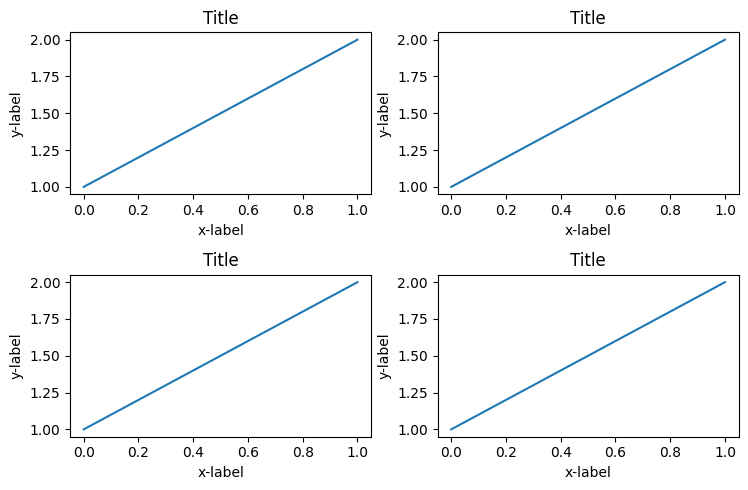Now you can see that the grids are adjusted perfectly so that there are no overlaps.

But then, there is repeating and redundant information if the charts share the same title, axis values.

To make the plots share a common X and Y axis, specify `sharex=True` and `sharey=True` in `plt.subplots()` when you create the figure and axes.

To remove the space between the plots altogether use `plt.subplots_adjust(wspace=0, hspace=0)`.

``````/* Plots that the X and Y axes */
import matplotlib.pyplot as plt
plt.rcParams.update({'figure.figsize':(7.5,5), 'figure.dpi':100})

def plotnew(ax):
ax.plot([1,2])

/* Plot */
fig, ((ax1, ax2), (ax3, ax4)) = plt.subplots(nrows=2, ncols=2, sharex=True, sharey=True)
fig.suptitle('Main Title')
plotnew(ax1)
plotnew(ax2)
plotnew(ax3)
plotnew(ax4)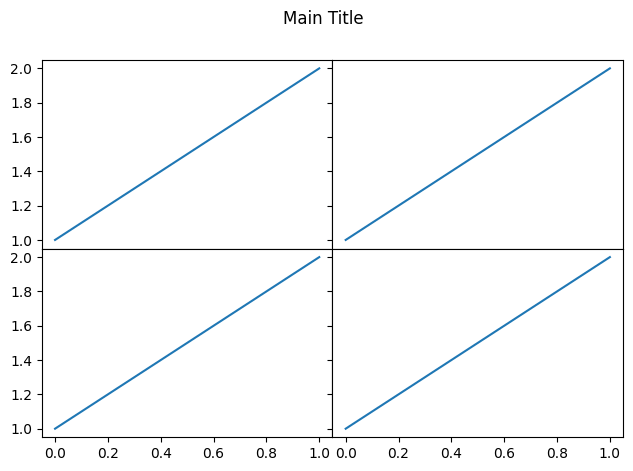## 14. Irregular plot layout using GridSpec

`plt.GridSpec()`is a great command if you want to create grids of different sizes in the same plot.

This can be used in a wide variety of cases for plotting multiple plots.

You need to specify the no of rows and no of columns as arguments to the fucntion along with the height and width space.

If you want to create a gridspec for a grid of two rows and two columns with some specified width and height space looks like this:

``grid = plt.GridSpec(2, 3, wspace=0.4, hspace=0.3)``

You will see that we can join 2 grids to form one big grid using the ,
operator inside the subplot() function.

``````plt.subplot(grid[0, 0])
plt.subplot(grid[0, 1:])
plt.subplot(grid[1, :2])
plt.subplot(grid[1, 2]);``````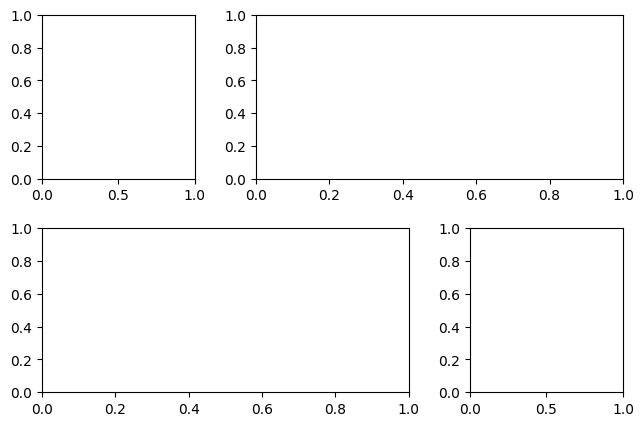# Special 95% discount

## 2000+ Applied Machine Learning & Data Science Recipes

### Portfolio Projects for Aspiring Data Scientists: Tabular Text & Image Data Analytics as well as Time Series Forecasting in Python & R## Two Machine Learning Fields

There are two sides to machine learning:

• Practical Machine Learning:This is about querying databases, cleaning data, writing scripts to transform data and gluing algorithm and libraries together and writing custom code to squeeze reliable answers from data to satisfy difficult and ill defined questions. It’s the mess of reality.
• Theoretical Machine Learning: This is about math and abstraction and idealized scenarios and limits and beauty and informing what is possible. It is a whole lot neater and cleaner and removed from the mess of reality.

Data Science Resources: Data Science Recipes and Applied Machine Learning Recipes

Introduction to Applied Machine Learning & Data Science for Beginners, Business Analysts, Students, Researchers and Freelancers with Python & R Codes @ Western Australian Center for Applied Machine Learning & Data Science (WACAMLDS) !!!

Latest end-to-end Learn by Coding Recipes in Project-Based Learning:

Applied Statistics with R for Beginners and Business Professionals

Data Science and Machine Learning Projects in Python: Tabular Data Analytics

Data Science and Machine Learning Projects in R: Tabular Data Analytics

Python Machine Learning & Data Science Recipes: Learn by Coding

R Machine Learning & Data Science Recipes: Learn by Coding

Comparing Different Machine Learning Algorithms in Python for Classification (FREE)

`Disclaimer: The information and code presented within this recipe/tutorial is only for educational and coaching purposes for beginners and developers. Anyone can practice and apply the recipe/tutorial presented here, but the reader is taking full responsibility for his/her actions. The author (content curator) of this recipe (code / program) has made every effort to ensure the accuracy of the information was correct at time of publication. The author (content curator) does not assume and hereby disclaims any liability to any party for any loss, damage, or disruption caused by errors or omissions, whether such errors or omissions result from accident, negligence, or any other cause. The information presented here could also be found in public knowledge domains.  `